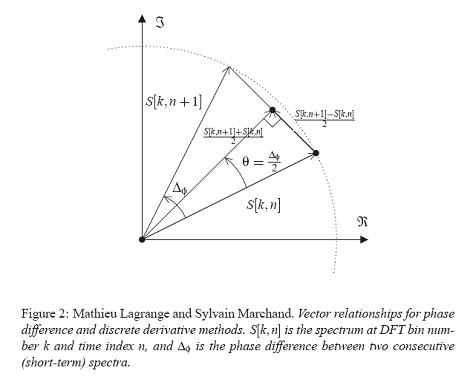## Estimation of the Instantaneous Frequency Using Phase-Based Methods

Associated People: Mathieu LagrangeThe robust estimation of the frequency of some sinusoidal components is a major prerequisite for many applications, such as in sinusoidal sound modeling, where the estimation has to be done with a low complexity, on short-term spectra. Among the estimators proposed in the literature, we focus in this paper on a class known as the "phase-based" estimators. In this study, we prove that five of these estimators are equivalent, at least in theory.

In-depth practical experiments demonstrate that these estimators perform roughly similarly in practice, although small differences remain, differences which are mainly due to numerical properties of the mathematical operators used in their implementations.

News

Latest Demos